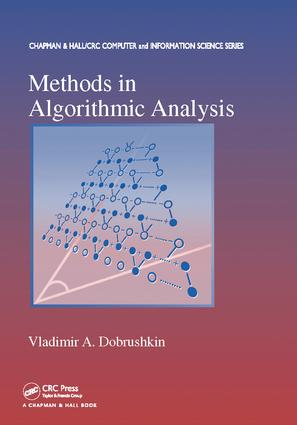# Methods in Algorithmic Analysis

## 1st Edition

Chapman and Hall/CRC

824 pages | 54 B/W Illus.

##### Purchasing Options:\$ = USD
Paperback: 9781138118041
pub: 2017-06-16
\$82.95
x
Hardback: 9781420068290
pub: 2009-11-03
Currently out of stock
\$220.00
x

FREE Standard Shipping!

### Description

Explores the Impact of the Analysis of Algorithms on Many Areas within and beyond Computer Science

A flexible, interactive teaching format enhanced by a large selection of examples and exercises

Developed from the author’s own graduate-level course, Methods in Algorithmic Analysis presents numerous theories, techniques, and methods used for analyzing algorithms. It exposes students to mathematical techniques and methods that are practical and relevant to theoretical aspects of computer science.

After introducing basic mathematical and combinatorial methods, the text focuses on various aspects of probability, including finite sets, random variables, distributions, Bayes’ theorem, and Chebyshev inequality. It explores the role of recurrences in computer science, numerical analysis, engineering, and discrete mathematics applications. The author then describes the powerful tool of generating functions, which is demonstrated in enumeration problems, such as probabilistic algorithms, compositions and partitions of integers, and shuffling. He also discusses the symbolic method, the principle of inclusion and exclusion, and its applications. The book goes on to show how strings can be manipulated and counted, how the finite state machine and Markov chains can help solve probabilistic and combinatorial problems, how to derive asymptotic results, and how convergence and singularities play leading roles in deducing asymptotic information from generating functions. The final chapter presents the definitions and properties of the mathematical infrastructure needed to accommodate generating functions.

Accompanied by more than 1,000 examples and exercises, this comprehensive, classroom-tested text develops students’ understanding of the mathematical methodology behind the analysis of algorithms. It emphasizes the important relation between continuous (classical) mathematics and discrete mathematics, which is the basis of computer science.

### Reviews

…helpful to any mathematics student who wishes to acquire a background in classical probability and analysis … This is a remarkably beautiful book that would be a pleasure for a student to read, or for a teacher to make into a year's course.

—Harvey Cohn, Computing Reviews, May 2010

PRELIMINARIES

Why Do We Analyze Algorithms?

Proofs

Iteration and Recursion

COMBINATORICS

Properties of Summation

Multiple Sums

Principles of Counting

Permutations and Combinations

Binomial Coefficients

Binomial Coefficient and Hypergeometric Functions

Stirling Approximation

PROBABILITY

Set Operations

Sample Space and Random Variables

Calculating Probabilities

Random Variables

Conditional Probabilities

Independence

Joint Distributions

Dependent Random Variables

Special Distributions

Types of Probabilistic Convergence

The Theorem of Total Probability

Bayes’ Theorem

Convolution

Order Statistics

Chebyshev Inequality

Sundry Examples

RECURRENCES OR DIFFERENCE EQUATIONS

How Do Difference Equations Arise?

Properties of Difference Equations

First Order Linear Difference Equations

Divide-and-Conquer Recurrences

Quicksort Recurrence

Recurrences in Numerical Analysis

Continued Fractions

Partial Difference Equations

Some Applications

INTRODUCTION TO GENERATING FUNCTIONS

Generating Functions—Definitions

Extraction of Coefficients

Counting Binary Trees

Solving Recurrences

Snake Oil Summation

Applications in Probability

The Langrage Inversion Theorem

ENUMERATION WITH GENERATING FUNCTIONS

Definition of Enumerators

Sum and Product Rules

Counting Compositions of Integers

Further Set Operations

Partition of Integers

Exponential Enumerators

FURTHER ENUMERATION METHODS

Enumeration of Trees

Occupancy Enumeration

The Principle of Inclusion and Exclusion (PIE)

Extensions and Further Applications of the PIE

Probabilistic Inclusion-Exclusion Principle

Runs in Permutations

Special Topics

COMBINATORICS OF STRINGS

Operations on Languages

Regular Languages

Counting Regular Languages

Waiting Time Probabilistic Problems

Algorithms and Markov Chains

INTRODUCTION TO ASYMPTOTICS

Asymptotic Notation and Applications

The Critical Range Method

Rice’s Method

The Euler Summation Formula

Finding Primes

Asymptotics from Recurrences

Limit Laws in Probability

ASYMPTOTICS AND GENERATING FUNCTIONS

Elementary Bounds from Generating Functions

Estimates from Singularities

Estimates from Entire Functions

Examples and Exercises

REVIEW OF ANALYTIC TECHNIQUES

Complex Numbers

Review of Power Series

Functions of a Complex Variable: Basic Concepts

Differential Operators

Partial Fraction Decomposition

Some Special Functions

Stieltjes Integrals

APPENDICES

BIBLIOGRAPHY

ANSWERS/HINTS TO SELECTED PROBLEMS

INDEX

### About the Author/Editor

Vladimir A. Dobrushkin is a professor in the Division of Applied Mathematics at Brown University and a professor in the Department of Computer Science at Worcester Polytechnic Institute.

### Subject Categories

##### BISAC Subject Codes/Headings:
COM000000
COMPUTERS / General
COM051300
COMPUTERS / Programming / Algorithms
COM059000
COMPUTERS / Computer Engineering
MAT000000
MATHEMATICS / General